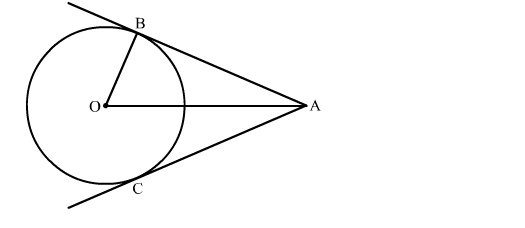# In the given figure, AB and AC are tangents to a circle with centre O and radius 8 cm.`
Question:

In the given figure, AB and AC are tangents to a circle with centre O and radius 8 cm. If OA = 17 cm, then the length of AC (in cm) is
(a) 9 cm
(b) 15 cm

(c) $\sqrt{353} \mathrm{~cm}$

(d) 25 cmSolution:

We know that the radius and tangent are perperpendular at their point of contact
In right triangle AOB
By using Pythagoras theorem, we have
OA2 = AB2 + OB2
⇒ 172 = AB2 + 82
289 = AB2 + 64
AB2 = 225
⇒ AB = 15 cm
The tangents drawn from the external point are equal.
Therefore, the length of AC is 15 cm
Hence, the correct answer is option (b).